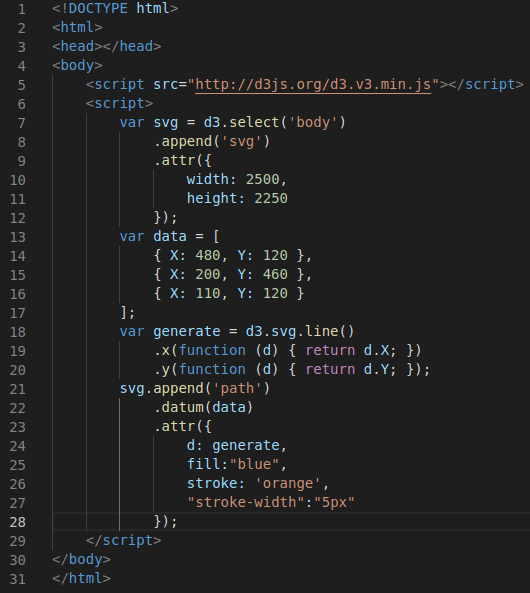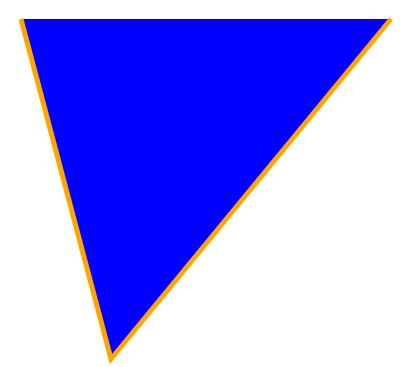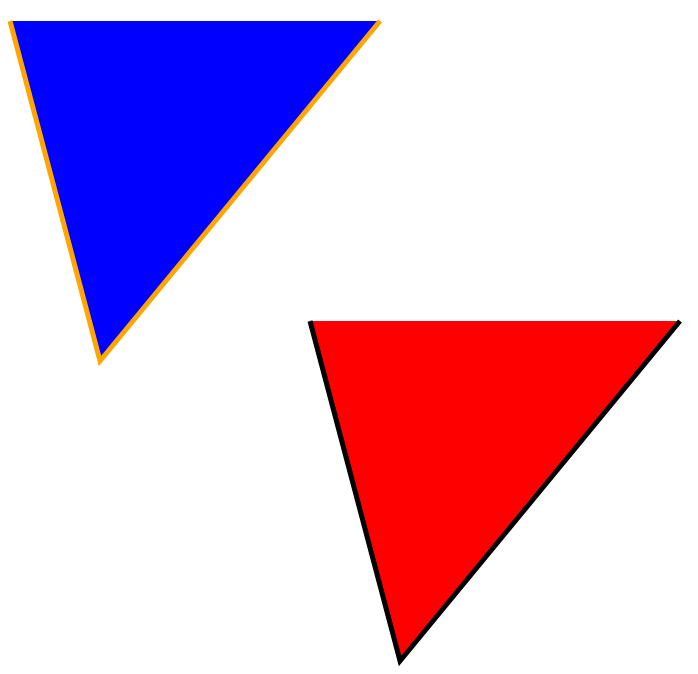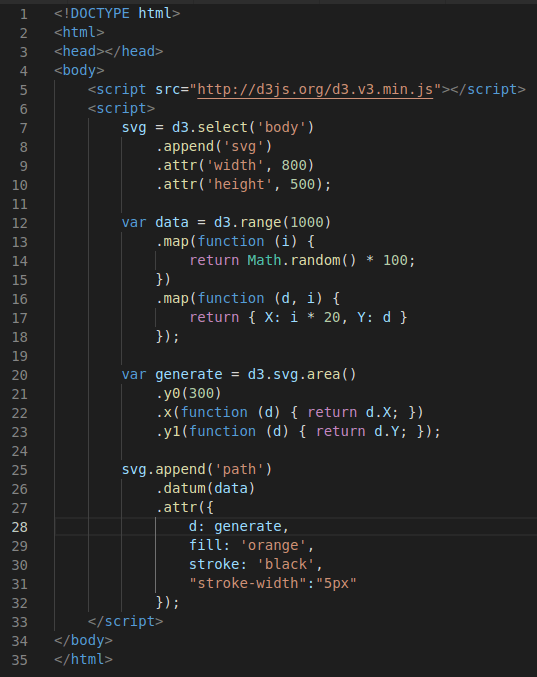# Path Generators in D3.js

D3.js has the ability to draw rather complex shapes using techniques called path generators. This will allow you to many things that would otherwise be difficult when working with visualizations.In this post, we will look at three different examples of path generation involving d3.js. The three examples are as follows

• Making a triangle
• Duplicating the triangle
• Make an area chart

Making a Triangle

To make a triangle we start by appending a svg to the body element of our document (lines 7-12). Then when create an array that has three coordinates. We need three coordinates because that is how many coordinates a triangle needs (lines 13-17). After this, we create a variable called generate and use the .svg.line() method to draw the actual lines that we want (lines 18-20).

After this, we append the data and other characteristics to the svg variable. This allows us to set the fill color and the line color (lines 21-28). Below is the code followed by the output.A simple triangle was created with only a few lines of code.

Create a Triangle and Duplicate

This example is an extension of the previous one. Here, we create the same triangle, but then we duplicate it using the translate method. This is a powerful technique if you need to make the same shape more than once.

The new code is found in lines 29-36You can see that the new triangle was moved to a different position based on the arguments we gave it.

Area Chart

Area charts are often used to make line plots but with the area under the line being filled with a certain color. To achieve this we do the following.

• Add our svg element to the body element (lines 7-10)
• Create some random data using the .range() method and several functions (lines 12-18)
• we generate 1000 random numbers (line 12) between 0 and 100 (line 14)
•   We set the Y values as random values of X in increments of 10 (line16-17)
• We create a variable called generate to create the path using the .area() method (line 20-23)
• y0 has to do with the height or depth of the area the other two attributes are the X and Y values
• We then append all this to the svg variable we created (lines 25-31)

Below is the code followed by the visual.Much more can be done with this but I think this makes the point for now.

Conclusion

This post was just  an introduction to making paths with D3.js.